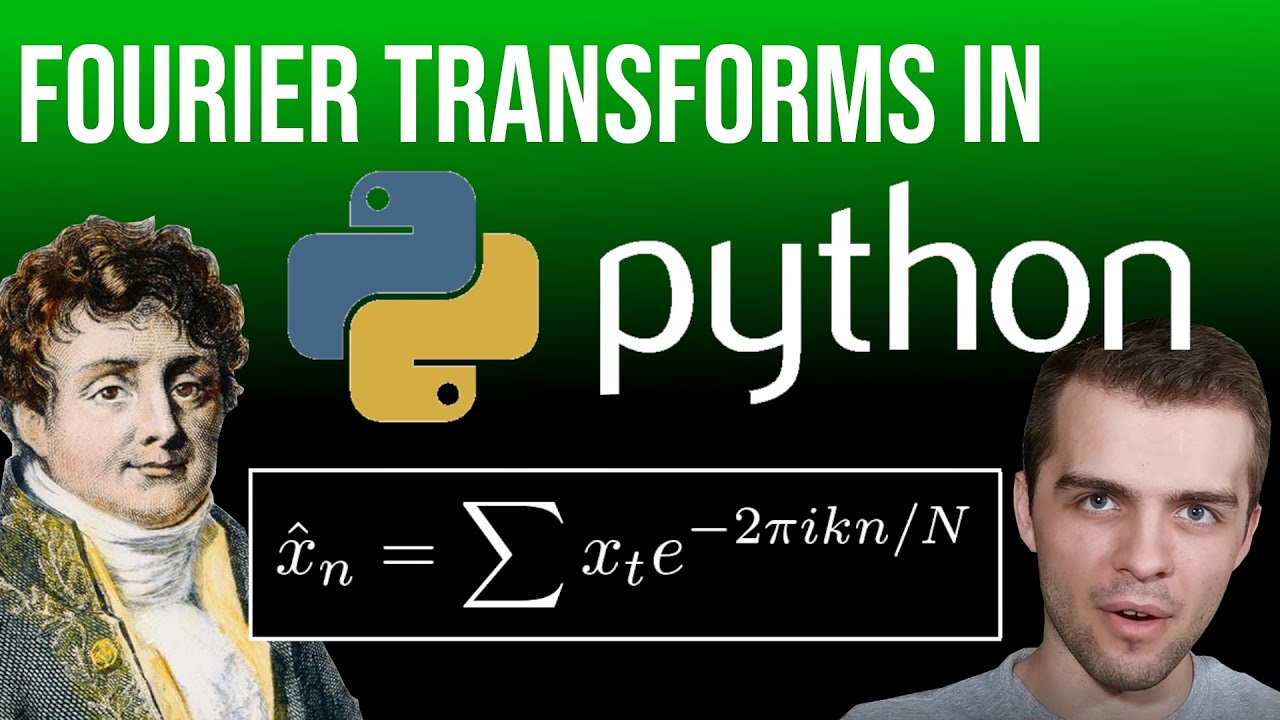# Top 3 Most Important Fourier Transforms in PythonIn this video I delve into the libraries of sympy and scipy to take a look at Fourier analysis in python. I look specifically at 1. Fourier Transforms, 2. Fourier Series, and 3. The Discrete Fourier Transform.

In this video I delve into the libraries of sympy and scipy to take a look at Fourier analysis in python. I look specifically at 1. Fourier Transforms, 2. Fourier Series, and 3. The Discrete Fourier Transform. Some of these transforms involve integrals that can be evaluated analytically (i.e. integrals can be solved symbolically using sympy) while other transforms involve integrals don’t have analytic solutions: numerical solutions can be obtained for such integrals using scipy.

Fourier Transforms show up in fields such as quantum mechanics when one wishes to convert a wavefunction in a position basis to a momentum basis. If the analytical form of the wavefunction is known, this transition is seamless using scipy or sympy. Fourier series can often show up in the study of partial differential equations. Finally, the discrete Fourier transform is a useful tool in data analysis to obtain a spectral density estimator for a given time series, for example.

2:46 1 .Fourier Transforms (Function Domain Unbounded)
8:35 2. Fourier Series (Function Domain Bounded)
15:05 3. Discrete Fourier Transform (Function Discretely Measured)